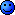# Education Help With Signal Analysis & Control

Discussion in 'General' started by Strudul, 7 May 2015.

1. ### Strudul~

Joined:
31 May 2010
Posts:
947
34
Hey

I'm trying to revise for my signal analysis and control exam, but I'm having trouble with a few of the questions. No matter how much I read through my notes and search on the internet, I can't work them out.

If anyone could explain them to me or at least point me in the right direction it would be greatly appreciated.

1a)I think I'm right in saying that Ta(s) / Vf(s) = K/ (1+Ts)
But then I have no idea how to calculate K.
For T, can I just say T = L/R (because it's an inductor)?

1b)Honestly not a clue where to start here...

2a)From what I can work out, the OLTF = G(s)H(s) and CLTF = [G(s)H(s)] / [1+ G(s)H(s)]
So, CLTF would be:?
Then I would need to rearrange it and sub the values of a,b and c in?
But then why am I told what the gain is?
Or am I completely off track and should be doing Laplace or something?

2b)Absolutely no idea and I'm assuming I need to know the answer to part a) to do it.

3a)3b)Really don't know.

Cheers

xTatic

2. ### UnicornUniform November India

Joined:
25 Jul 2006
Posts:
12,726
456
I'll have a look through my notes when I get a few minutes, I studied a module similar to that in Mechatronics. What year are you in?

3. ### Strudul~

Joined:
31 May 2010
Posts:
947
34
2nd Year

In 1st year we were given past questions and solutions that I could work through, but this year they are refusing to provide them for some reason and it's making it so much harder :/

4. ### UnicornUniform November India

Joined:
25 Jul 2006
Posts:
12,726
456
Yeah I think I took that in 2nd year as well. I'll try to help but it's been a while! I tend to forget a lot of theory the week after the exam :/

5. ### Strudul~

Joined:
31 May 2010
Posts:
947
34
Better then than before... or during6. ### SkiDaveMinimodder

Joined:
16 Mar 2008
Posts:
724
47
I can't remember how to do q1.

q2 a:
CLTF = [G_c(s)G_c(s)H(s)] / [1+ G_c(s)G_c(s)H(s)]

= [k_p / (s+a)(s+b)(s+c)] / [1+ k_p / (s+a)(s+b)(s+c)]

q2b:
You need to get the Closed Loop Characteristic Equation (CLCE). As the question hasn't said otherwise you can assume unity H(s). ie H(s)=1

CLCE: 1+ G_c(S)G_p(s)

= 1+ k_p / (s+a)(s+b)(s+c)

sub in the values and rearrange into a cubic.
They gave you one of the roots so that you only have to find the roots of a quadratic.

I can't remember how to prove stability. I'll have another look at my notes and get back to you

EDIT: To show stability all the roots of the CLCE have to have negative real parts

Last edited: 7 May 2015
7. ### SkiDaveMinimodder

Joined:
16 Mar 2008
Posts:
724
47
For q3a:

The steady state error is given by:

e = r / [1 + G(0)]

In this case r=1 as it is a unit step.

so
e = 1 / [1 + k.G(0)]

q3b:

you have to show the e = 0 when

e = 1 / [1 + (k + ki/s).G(s)] when s=0

8. ### Strudul~

Joined:
31 May 2010
Posts:
947
34
Thanks Dave. Really helped.

2a) That seems like a very easy 7 marks.

2b) Rearranging and subbing the values, I got:
s^3 + 8s^2 + 6s + 260 = 0
(s+10)(s^2 -2s + 26) = 0

(s^2 -2s +26) only has imaginary roots, therefore the system is unstable.

3a) Subbing s = 0 into the OLTF gave G(0) = A.
Which fit nicely to give the correct error.

3b) I'm still kinda stuck on how to prove this one.
Am I okay to use my result from part a) and sub G(s) = A?
Giving 1/ [1 + (K+ Ki/s)A]
But then I have something divided by s, which would be divided by 0, which I can't do....
I tried rearranging, multiplying and factoring things, but I couldn't seem to be able to get it to a point where i can sub s=0 in.
What am I missing here?

Last edited: 7 May 2015